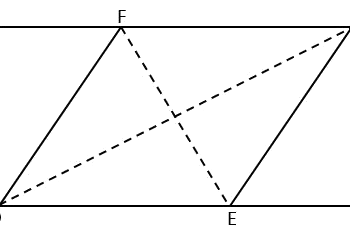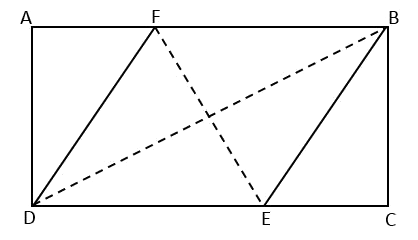## Challenging Questions on Various Secondary Math Topics

### Challenging Questions on Various Secondary Math Topics

I have compiled some of the challenging questions I have covered with my students. For those who are interested, you are more than welcomed to give the questions below a try! Cheers =) .
$$\\[1pt]$$

QUESTIONS:

$${\small 1.\enspace}$$ Given that:
$$\\[1pt]$$
$${\LARGE\frac{108}{17} \ = \ } {\LARGE a} + \huge{\frac{1}{b \ + \ \frac{1}{c \ + \ \frac{1}{d \ + \ 2}}}}$$
$$\\[1pt]$$
with a, b, c and d are positive integers. Find the value of:
$$\\[1pt]$$
$${\LARGE\frac{a^3 \ – \ 3a^2b \ + \ 3ab^2 \ – \ b^3}{c^3 \ – \ 3c^2d \ + \ 3cd^2 \ – \ d^3}}$$

$$\\[1pt]$$
$${\small 2.\enspace}$$ One year, 23 September was a Monday. What day of the week was 10 November that year?
$$\hspace{1.2em}$$(a). Sunday
$$\hspace{1.2em}$$(b). Monday
$$\hspace{1.2em}$$(c). Thursday
$$\hspace{1.2em}$$(d). Saturday

$$\\[1pt]$$
$${\small 3.\enspace}$$ In a rectangle ABCD, point F and E are located at the side AB and DC in such a way a rhombus BFDE is formed, as shown in the picture.If AB $$=$$ 6.4 cm and BC $$=$$ 4.8 cm, find EF.

$$\\[1pt]$$
$${\small 4.\enspace}$$ Given that: $$(3x\:+\:4y \:-\: 5z)^{3}$$, find the sum of coefficients of the terms: $$x^2y,\:y^2z$$ and $$x^2z$$.

$$\\[1pt]$$
$${\small 5.\enspace}$$ Find the result of:
$$\\[1pt]$$
$${\large\frac{5}{(2 \ \times \ 3)^{2}}}+{\large\frac{7}{(3 \ \times \ 4)^{2}}}+{\large\frac{9}{(4 \ \times\ 5)^{2}}} + \dots + {\large\frac{23}{(11 \ \times \ 12)^{2}}}$$

$$\\[1pt]$$
$${\small 6.\enspace}$$ Mr. Santoso has five kids. Each of them is given a different amount of pocket money per week. Adi gets half of what Edi receives, Beni gets $1 more from Adi, Citra gets$1.5 more than Adi and Doni gets $1.7 less that Edi. If the average of their pocket money is$5.2 in a week, what is the amount of pocket money that Doni received in a year?

$$\\[1pt]$$
$${\small 7.\enspace}$$ The same rule is applied to the top number in each box to give the bottom number.
$$\\[1pt]$$$$\\[1pt]$$
What number must box x be ?
(a). 30
(b). 32
(c). 34
(d). 40

$$\\[1pt]$$
$${\small 8.\enspace}$$ Oscar, Lily and Jack collect souvenir coins. Oscar has 44 more coins than Lily and 48 more coins than Jack. Oscar has 6 more coins than Lily and Jack combined. How many coins do Oscar, Lily and Jack have altogether?
(a). 196
(b). 166
(c). 156
(d). 146

$$\\[1pt]$$
$${\small 9.\enspace}$$ Sam had identical copies of these three paper shapes.
$$\\[1pt]$$$$\\[1pt]$$
He used them to create these designs.
$$\\[1pt]$$$$\\[1pt]$$
What value must x be?
(a). 10
(b). 11
(c). 12
(d). 13

$$\\[1pt]$$
$${\small 10.\enspace}$$ In the equation below, A and B represent natural numbers. What values of A and B will make the equation true?
$$\\[1pt]$$
$${\large \frac{A}{3} \ + \ \frac{B}{4} \ = \ \frac{11}{12}}$$

$$\\[1pt]$$

As always, if you have any particular questions to discuss, leave it in the comment section below. Cheers =) .